# Team:Grenoble-EMSE-LSU/Project/Validation

Grenoble-EMSE-LSU, iGEM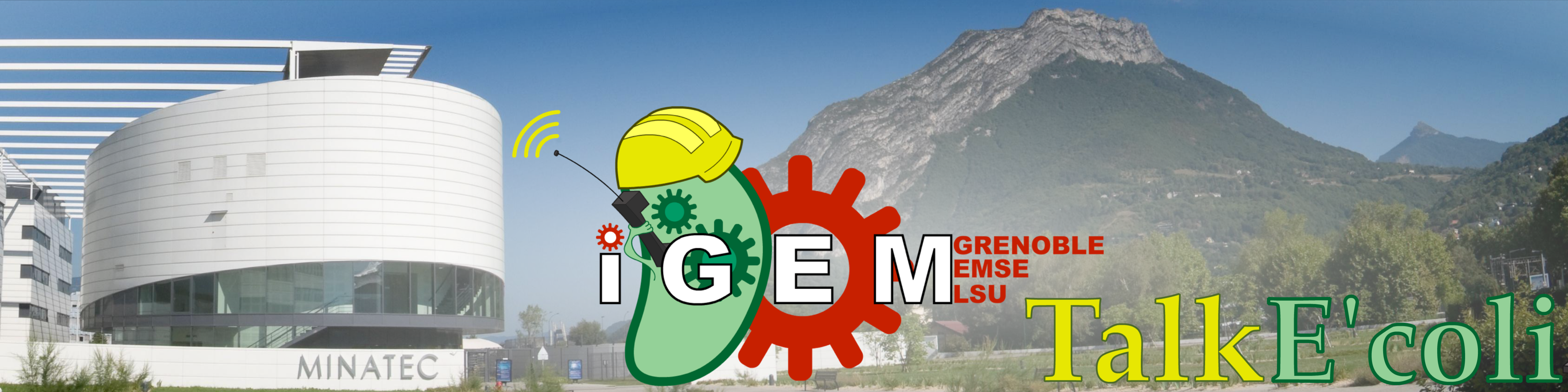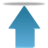Grenoble-EMSE-LSU, iGEM

• # Validation

To validate our heuristic method, we ran two experiments where we tried to stabilize the concentration of living cells in a bacterial culture during 4 consecutive hours. Both were successful, and we will report in more detail one of them.

• # Experiment

In the first part of the experiment, we first determined the parameters of the model that describe the behavior of our cell culture, according to the procedure described in the modeling part.

On the curves below:

$\bullet$ The red surface is the predicted absorbance of dead bacteria ($D$, in $OD_{600}$) in the absorbance panel, and the predicted KillerRed fluorescence in dead bacteria ($K_D$, in $RFU$) in the fluorescence panel.

$\bullet$ The yellow surface represents the predicted absorbance of living bacteria ($C$, in $OD_{600}$) in the absorbance panel, and the predicted KillerRedfluorescence KillerRed stored in living bacteria ($K$, in $RFU$) in the fluorescence panel.

$\bullet$ The blue line represents experimental datas.

Step 1 ($t=330min$):

This step is divided in two periods : first 1.5 hours in the dark, then 4 hours at maximal intensity of the lamp ($I = 1$). This procedure has 2 objectives : to obtain a precised model and to reach more rapidly the desired density of living bacteria. A first estimate of the cell culture parameters were determined by fitting the curves obtained so far. We then searched for the light profile that would stabilize the cell population. Light intensity is expressed as a fraction or a percentage of the maximum intensity of the bulb. A constant 30% light level could stabilize the living cell density, but it took to much time. We therefore decided to illuminate first at 70% for 2 hours, then to decrease the intensity to 30%. Below are shown the fit of the 5 first hours and the predicted $OD_{600}$ and fluorescence for the upcoming intensities. The cell density should reach its target level (0.02) after 2 hours.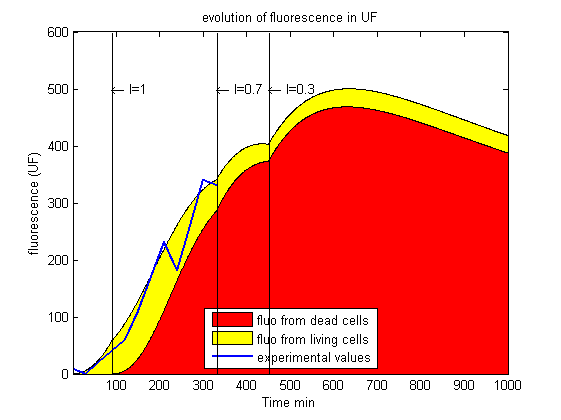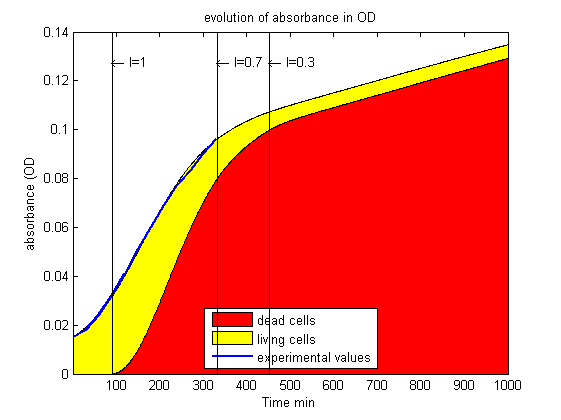Parameters for these predictions:

$R=83 min$

$a=140 RFU.OD^{-1}.min^{-1}$

$b=0,9.10^{-2}RFU.UL^{-1}.min^{-1}$

$k=0,9.10^{-7}OD.RFU^{-1}.UL^{-1}.min^{-1}$

$l=0.983$

$M=110 min$

Step 2 ($t=450min$):

After 2 hours, we observe that the fluorescence has closely followed the prediction, but that the absorbance has grown up too fast. The model parameters are thus adjusted again and therefore the predicted kinetics are re-calculated. This may force us to change the light intensity time profile.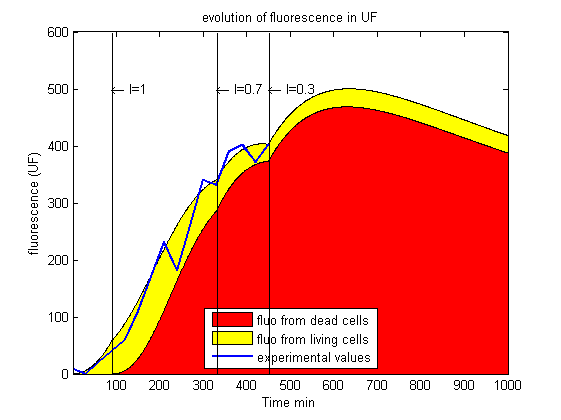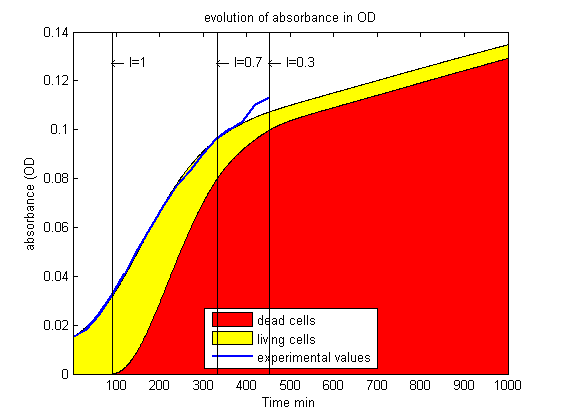Step 3:

Here are the newly predicted kinetics, with the new set of parameters. It seems that an 30% illumination may be insufficient to stabilize the bacterial population.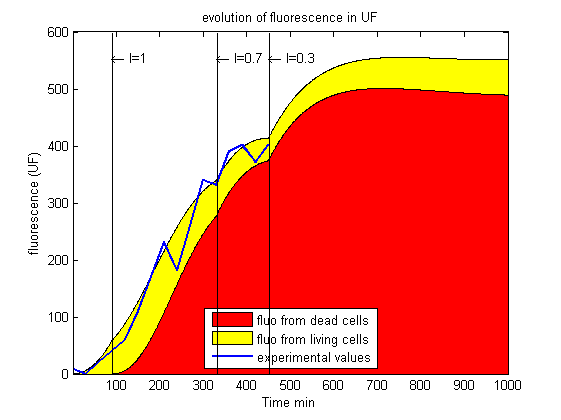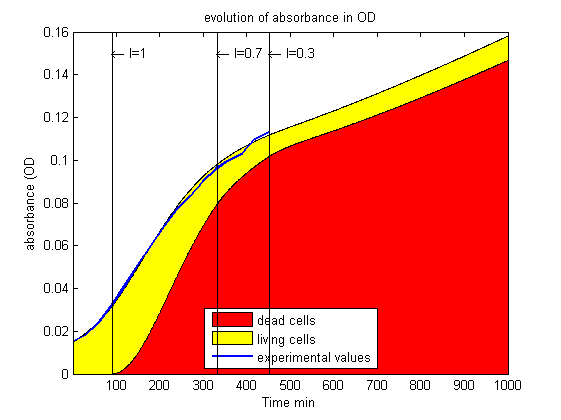Parameters for these predictions:

$R=83 min$

$a=140 RFU.OD^{-1}.min^{-1}$

$b=0,93.10^{-2}RFU.UL^{-1}.min^{-1}$

$k=0,93.10^{-7}OD.RFU^{-1}.UL^{-1}.min^{-1}$

$l=0.9811$

$M=110 min$

Step 4:

The light intensity has to be increased a little bit. Again, to reach the target density more rapidly, we chose a 50% illumination for 2 hours, followed by a 30% illumination. Below are shown the predicted kinetics.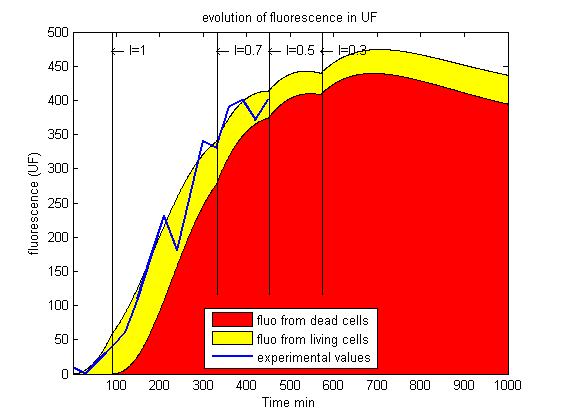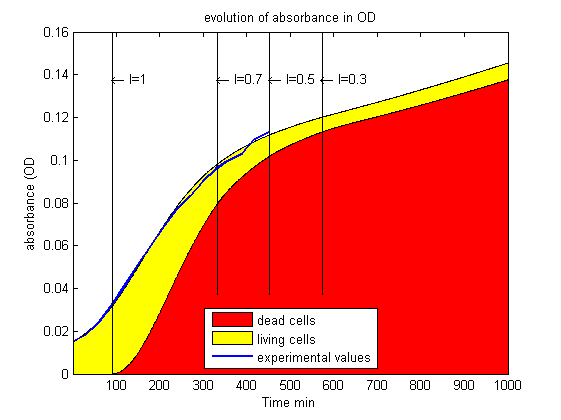Step 5 ($t=570min$):

This time, the absorbance fits perfectly the predicted curve, but the fluorescence does not. It instead decreased faster than expected. Just as in 'step 2', the parameters have to be adjusted to improve the fit.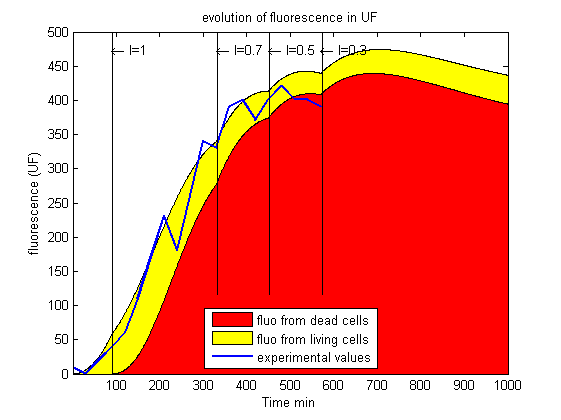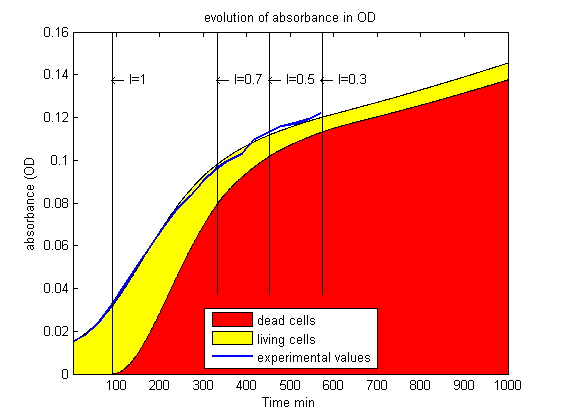Step 6:

Here are the data fitted with the improved set of parameters. Again, the illumination profile needed to be adjusted. 30% illumination was not enough and we increased it to 40%.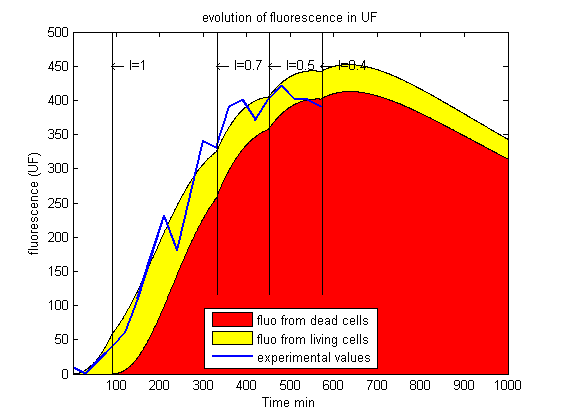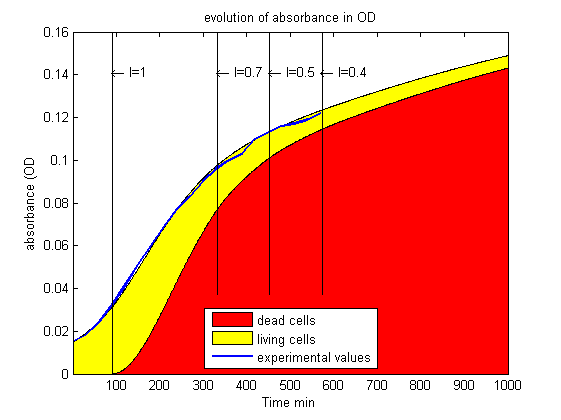Parameters for these predictions:

$R=84.5 min$

$a=140 RFU.OD^{-1}.min^{-1}$

$b=1.0.10^{-2}RFU.UL^{-1}.min^{-1}$

$k=0,9.10^{-7}OD.RFU^{-1}.UL^{-1}.min^{-1}$

$l=0.9807$

$M=110 min$

Step 7:

The OD curve is satisfactory, since a 4-hours long linear increase of absorbance can be observed (from $t=360min$ to $t=630min$). This suggests that our procedure works. We still have to compare our results to the number of bacterial colonies able to grow on Petri dishes.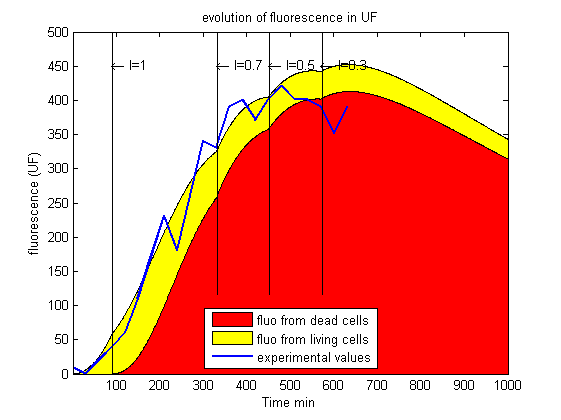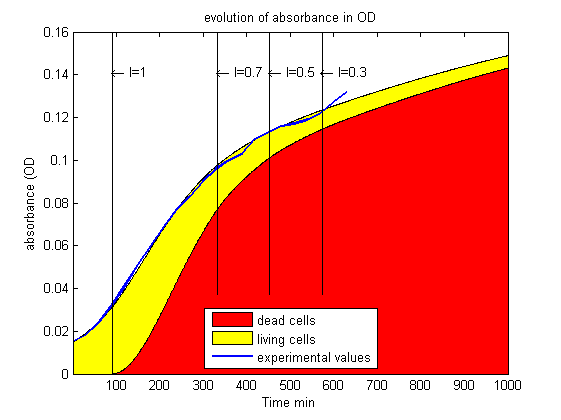In the final absorbance curve, the optical density of living cell is about 0.02 ($OD_{600}$). Considering that a cell concentration of $3.10^5 cells/μL$ result in an absorbance of $1 OD_{600}$, we thus expect a colony forming unit concentration of $6000 cells/μL$.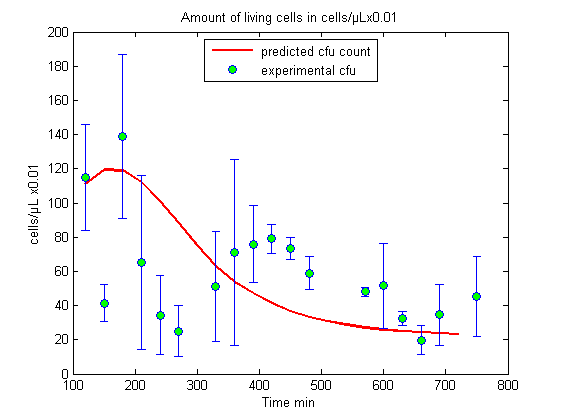And this is exactly what we obtained (in average).

We have been able to control a bacterial population for 4 consecutive hours .